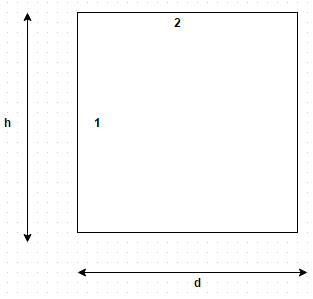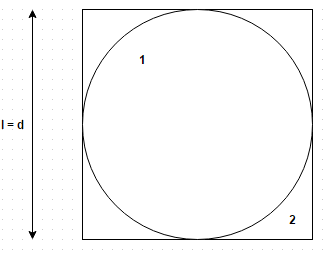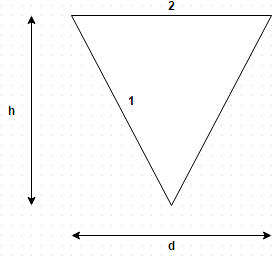Courses

# Test: Shape Factor

## 11 Questions MCQ Test Heat Transfer | Test: Shape Factor

Description
This mock test of Test: Shape Factor for Chemical Engineering helps you for every Chemical Engineering entrance exam. This contains 11 Multiple Choice Questions for Chemical Engineering Test: Shape Factor (mcq) to study with solutions a complete question bank. The solved questions answers in this Test: Shape Factor quiz give you a good mix of easy questions and tough questions. Chemical Engineering students definitely take this Test: Shape Factor exercise for a better result in the exam. You can find other Test: Shape Factor extra questions, long questions & short questions for Chemical Engineering on EduRev as well by searching above.
QUESTION: 1

### Which of the followings is a wrong statement? The shape factor is equal to one

Solution:

For a flat or convex surface, the shape factor with respect to itself is zero. This aspect stems from the fact that for any part of flat or convex surface, one cannot see any other part of the same surface.

QUESTION: 2

### Establish a relation for the shape factor of cylindrical cavity with respect to itself of depth h and diameter d. The cavity is closed on its outer surface with a flat plateSolution:

11 + F 12 = 1 and F 21 + F 22 = 1. But F 22 = 0, so F 11 = 1 – A / A 1.

QUESTION: 3

### The reciprocity theorem states that Where symbols have their usual meanings

Solution:

It indicates the net radiation exchange can be calculated by computing one way configuration factor from either surface to the other.

QUESTION: 4

Two radiating surface A 1 = 6 m2 and A 2 = 4 m2 have shape factor F 12 = 0.1. Then the shape factor F 21 will be

Solution:

12 = A 2 F 21.

QUESTION: 5

What is the value of shape factor for two infinite parallel surfaces separated by a distance x?

Solution:

All the radiation emitted by one falls on the other so shape factor is unity.

QUESTION: 6

What is the shape factor of a sphere of diameter d inside a cubical box of length l = d?Solution:

11 + F 12 = 1 or F 12 = 1. So, F 21 = π/6.

QUESTION: 7

What is the shape factor of hemispherical surface closed by a plane surface of diameter d?

Solution:

11 + F 12 = 1 and F 21 = 1. Therefore, F 11 = 0.5.

QUESTION: 8

What is the shape factor of hemispherical surface closed by a plane surface of diameter d?

Solution:

11 + F 12 = 1 and F 21 = 1. Therefore, F 11 = 0.5.

QUESTION: 9

Establish a relation for shape factor for a conical cavity with respect to itself of depth h and diameter d. The cavity is closed on its outer surface with a flat plateSolution:

11 = 1 – A / A 1 = 1 – 2 sin α = 1 – d/ (4 h 2 + d 2½.

QUESTION: 10

What is the shape factor for a hemispherical bowl with respect to itself of diameter d? The cavity is closed on its outer surface with a flat plate

Solution:

11 = 1 – A / A = 0.5.

QUESTION: 11

Consider a system of concentric spheres of radius r 1 and r 2 (r 2 is greater than r 1). If r 1 = 5 cm, determine the radius r 2 if it is desired to have the value of shape factor F 21 equal to 0.6

Solution:

From reciprocity theorem, A 12 = A 2 F 21.